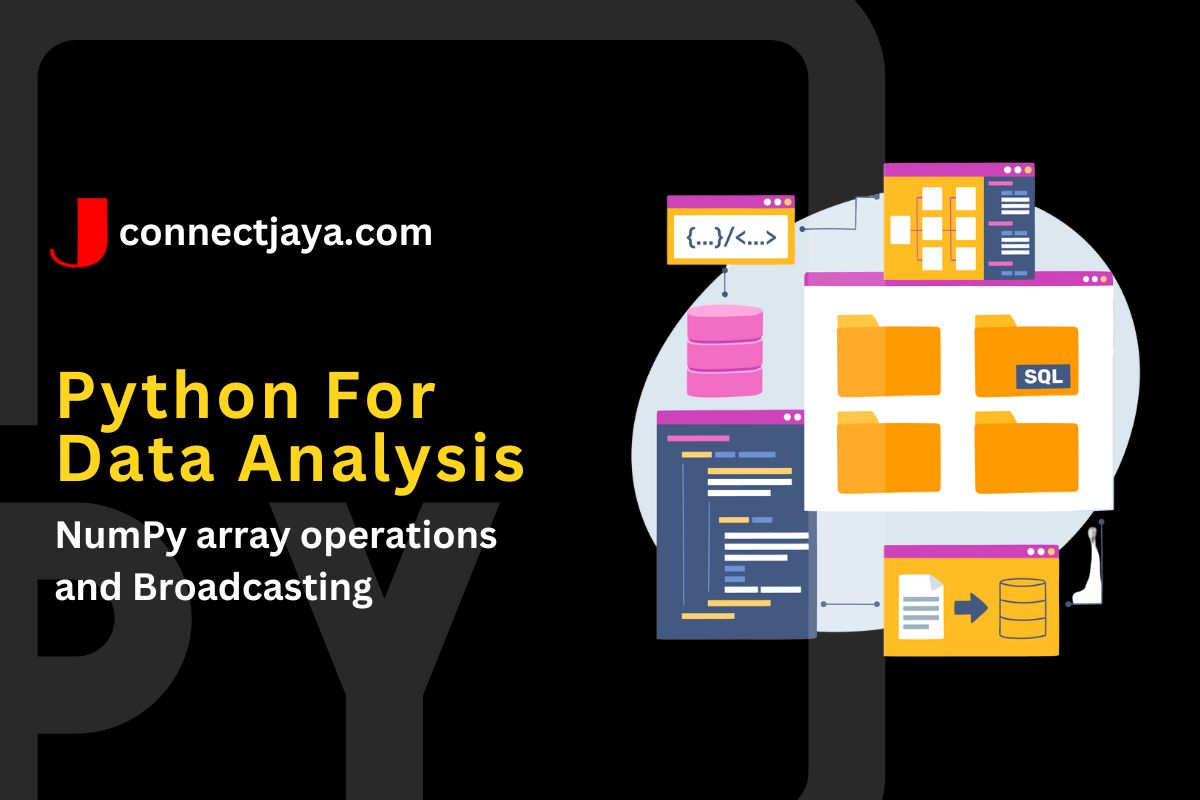## NumPy array operations and Broadcasting

NumPy provides a wide range of operations that can be performed on arrays, including mathematical, logical, and relational operations. These operations can be used to manipulate data in a variety of ways, such as filtering, transforming, and aggregating data.

One of the key features of NumPy is its ability to perform broadcasting. Broadcasting allows NumPy to perform operations on arrays of different shapes and sizes, without the need for explicit loop structures. Broadcasting allows for more concise and readable code, and can often result in improved performance.

### Here are some examples of NumPy array operations and broadcasting:

#### Mathematical operations:

import numpy as np

# Create two arrays
a = np.array([1, 2, 3])
b = np.array([4, 5, 6])

c = a + b
print(c)

# Subtract two arrays element-wise
d = a - b
print(d)

# Multiply two arrays element-wise
e = a * b
print(e)

# Divide two arrays element-wise
f = a / b
print(f)


#### Logical operations

import numpy as np

# Create an array
a = np.array([True, False, True])

# Invert the elements of the array
b = np.invert(a)
print(b)

# Combine two arrays using the logical AND operator
c = np.logical_and(a, b)
print(c)

# Combine two arrays using the logical OR operator
d = np.logical_or(a, b)
print(d)


#### Relational operations:

import numpy as np

# Create two arrays
a = np.array([1, 2, 3])
b = np.array([2, 3, 4])

# Check if the elements of the arrays are equal
c = np.equal(a, b)
print(c)

# Check if the elements of the arrays are not equal
d = np.not_equal(a, b)
print(d)

# Check if the elements of the first array are less than the elements of the second array
e = np.less(a, b)
print(e)


import numpy as np

# Create an array
a = np.array([1, 2, 3])

# Add a scalar value to each element of the array
b = a + 1
print(b)

# Add two arrays of different shapes
c = a + np.array([1, 2])
print(c)

# Multiply two arrays of different shapes
d = a * np.array([, , ])
print(d)


### Usecase

NumPy array operations and broadcasting are widely used in various data analysis use cases, such as:

1. Image processing: NumPy arrays are often used to represent digital images, which are typically represented as multi-dimensional arrays of pixel values. NumPy’s mathematical and logical operations can be used to perform various image processing tasks, such as filtering, smoothing, and edge detection.
2. Scientific computing: NumPy arrays are widely used in scientific computing tasks, such as numerical simulations and data analysis in fields such as physics, chemistry, and biology. NumPy’s mathematical operations and broadcasting capabilities can be used to perform various numerical computations, such as solving equations and simulating complex systems.
3. Machine learning: NumPy arrays are the basis of many popular machine learning libraries, such as Scikit-learn, Keras, and TensorFlow. NumPy’s mathematical and logical operations are used extensively in these libraries to perform various data preprocessing and transformation tasks, such as feature scaling and normalization.
4. Financial analysis: NumPy arrays can be used to represent and analyze financial data, such as stock prices and market trends. NumPy’s mathematical operations and broadcasting capabilities can be used to perform various financial calculations, such as calculating moving averages and identifying trading signals.
5. Data visualization: NumPy arrays can be used to create visualizations of data, such as scatter plots, histograms, and heat maps. NumPy’s broadcasting capabilities can be used to perform various transformations on data before visualizing it, such as scaling and normalization.

These are just a few examples of the many ways that NumPy array operations and broadcasting can be used in data analysis. NumPy’s flexibility and power make it an essential tool for anyone working with data in Python.